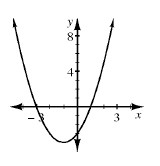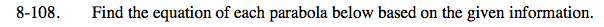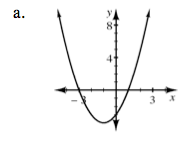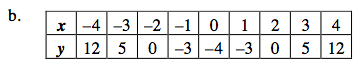### Home > CAAC > Chapter 8 > Lesson 8.3.2 > Problem8-108

8-108.
1. Find the equation of each parabola below based on the given information. Homework Help ✎

1.2.  x −4 −3 −2 −1 0 1 2 3 4 y 12 5 0 −3 −4 −3 0 5 12x-intercepts at (−3,0) and (1,0).

x = −3 or x = 1
x + 3 = 0 or x − 1 = 0
(x + 3)(x − 1) = 0

At the x-intercept, y = 0.

y = (x + 3)(x − 1) or y = x² +2x − 3

Use the same strategy as part (a) to solve part (b).